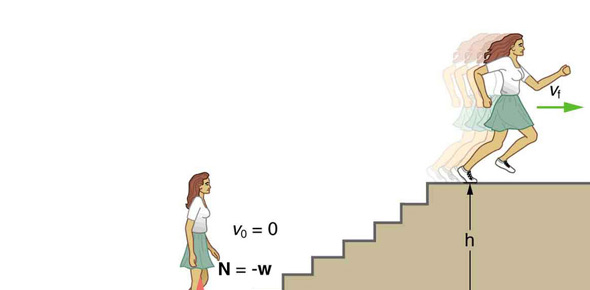# Quiz #5: Work, Power And Energy

10 Questions | Attempts: 895
ShareSettingsYou may take this quiz 3 times. I will keep your best score.

• 1.
If a mass is at rest, what must be applied to cause it to move?
• A.

Kilograms

• B.

Speed

• C.

Vectors

• D.

Force

• 2.
If you push on a wall with a force of 30 N, then ______________.
• A.

The wall pushes on you with a force of 30 N.

• B.

The wall moves back 3 cm.

• C.

The wall cannot be forced.

• D.

You are pulled towards the wall.

• 3.
If you have to push a car forward, what are the forces acting on you?
• A.

The car pushes back on you, gravity pulls you down, the ground pushes up on you, and friction on your shoes pushes forward on you.

• B.

Only gravity.

• C.

Your own muscles push you down.

• D.

No forces act on you.

• 4.
A person jumps to the ground from a platform 10 feet high.  Which of the following is true?
• A.

The person has more kinetic energy before they jump than after they jump.

• B.

The person has less potential energy at the top of the platform than in the air.

• C.

The person's potential energy at the instant they jump is approximately equal to their kinetic energy the instant they land.

• D.

The person's kinetic energy at the instant they jump is approximately double their potential energy the instant they land.

• 5.
The potential energy of an object is equal to ____________.
• A.

The work done on the object to put the object into its current state.

• B.

The velocity of the object divided by its mass.

• C.

The force of gravity working on the object.

• D.

The size of the object compared to its surface area.

• 6.
The kinetic energy of an object will increase the most if you __________________.
• A.

Double the velocity of the object

• B.

Double the mass of the object

• C.

Double the potential energy

• D.

Double the temperature of the object

• 7.
"Power" is defined as _________________.
• A.

The rate at which work is done

• B.

The energy necessary to do work

• C.

The velocity of an object squared

• D.

The sum of potential and kinetic energy

• 8.
An object with mass "m" kilograms is suspended "h" meters above the ground by a string.  If the string is cut, what will the velocity of the object be at the instant it hits the ground?
• A.

The square root of 2gh

• B.

One-half the mass squared

• C.

Two times ghm squared

• D.

H-2gm

• 9.
Which of the following is NOT a consequence of "The Law of Conservation of Energy"?
• A.

Potential Energy can never be greater than Kinetic Energy

• B.

The energy in a closed system is always constant

• C.

The Kinetic Energy of a dropped object at the instant it hits the ground is roughly equal to the potential energy of the object at the instant it was dropped

• D.

It is not necessary to use Kinematics to discover the velocity of an object accelerated by gravity if you know it's initial Potential Energy and its mass.

• 10.
As an object goes up a hill at constant velocity, which of the following is NOT true?
• A.

The object's Potential Energy is increasing.

• B.

The object's Kinetic Energy is decreasing.

• C.

The object's Kinetic Energy is constant.

• D.

The object's speed is constant.

## Related TopicsBack to top
×

Wait!
Here's an interesting quiz for you.